# How to Calculate and Solve for Petrosky-Farshad Gas Solubility in a Fluid | The Calculator Encyclopedia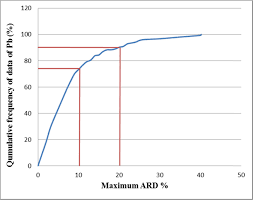The image above represents petrosky-farshad gas solubility.

To compute for the petrosky-farshad gas solubility, three essential parameters are needed and these parameters are gas specific gravity (γg), petrosky-farshad gas solubility parameter (x) and pressure (P).

The formula for calculating the petrosky-farshad gas solubility:

Rs = [(P/112.727 + 12.340)γg0.8439 10x]1.7384

Where:

γg = Gas Specific Gravity
x = Petrosky-Farshad Gas Solubility Parameter
P = Pressure

Let’s solve an example;
Find the petrosky-farshad gas solubility when the gas specific gravity is 16, the petrosky-farshad gas solubility parameter is 22 and the pressure is 80.

This implies that;

γg = Gas Specific Gravity = 16
x = Petrosky-Farshad Gas Solubility Parameter = 22
P = Pressure = 80

Rs = [(P/112.727 + 12.340)γg0.8439 10x]1.7384
Rs = [(80/112.727 + 12.340)160.8439 x 1022]1.9384
Rs = [(0.709 + 12.340)160.8439 x 1022]1.9384
Rs = [(13.049)160.8439 x 1022]1.9384
Rs = [(13.049)10.379 x 1022]1.9384
Rs = [1.354e+24]1.9384
Rs = 8.925e+41

Therefore, the Petrosky-Farshad gas solubility is 8.925e+41.

Nickzom Calculator – The Calculator Encyclopedia is capable of calculating the petrosky-farshad gas solubility.

To get the answer and workings of the petrosky-farshad gas solubility using the Nickzom Calculator – The Calculator Encyclopedia. First, you need to obtain the app.

You can get this app via any of these means:

To get access to the professional version via web, you need to register and subscribe for NGN 1,500 per annum to have utter access to all functionalities.
You can also try the demo version via https://www.nickzom.org/calculator

Apple (Paid) – https://itunes.apple.com/us/app/nickzom-calculator/id1331162702?mt=8
Once, you have obtained the calculator encyclopedia app, proceed to the Calculator Map, then click on Petroleum under EngineeringNow, Click on Fluid Properties under Petroleum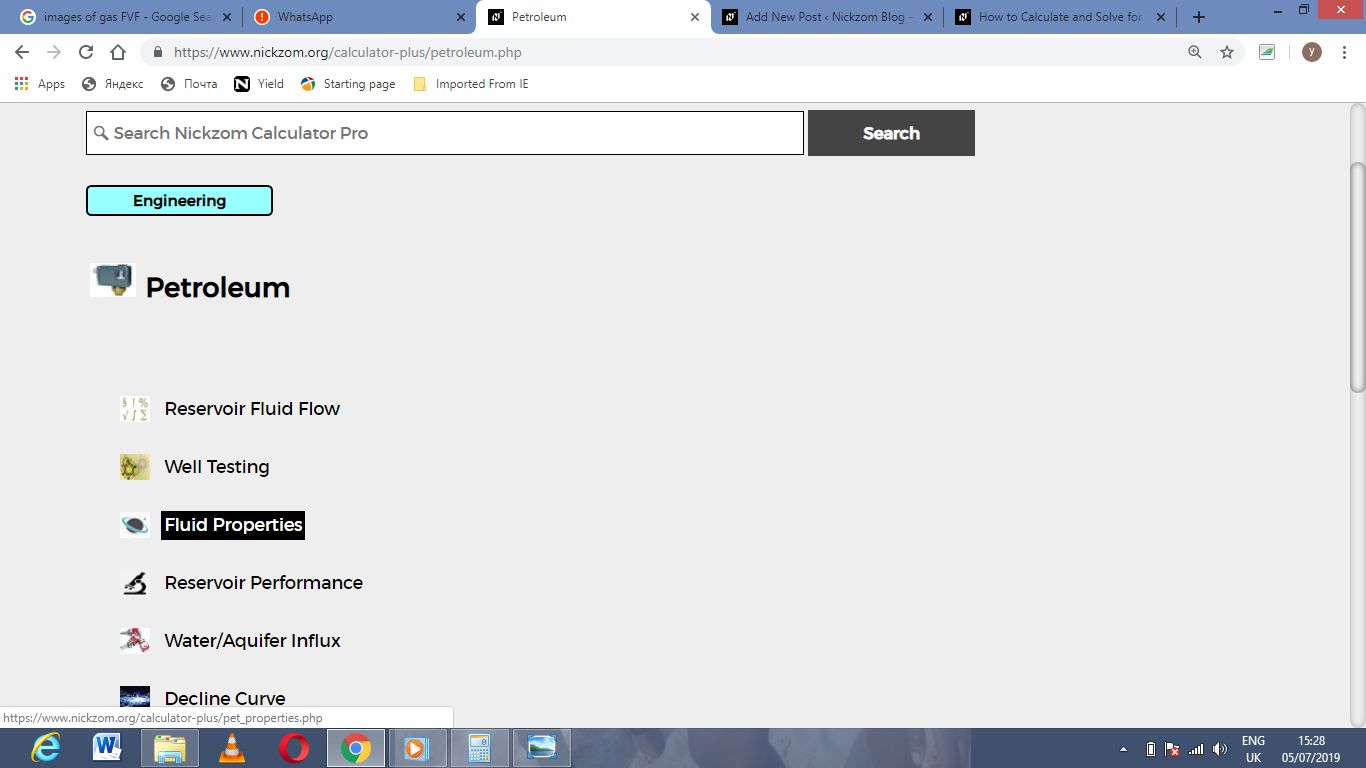Now, Click on Petrosky-Farshad Gas Solubility under Fluid PropertiesThe screenshot below displays the page or activity to enter your values, to get the answer for the petroksy-farshad gas solubility according to the respective parameters which are the gas specific gravity (γg), petrosky-farshad gas solubility parameter (x) and pressure (P).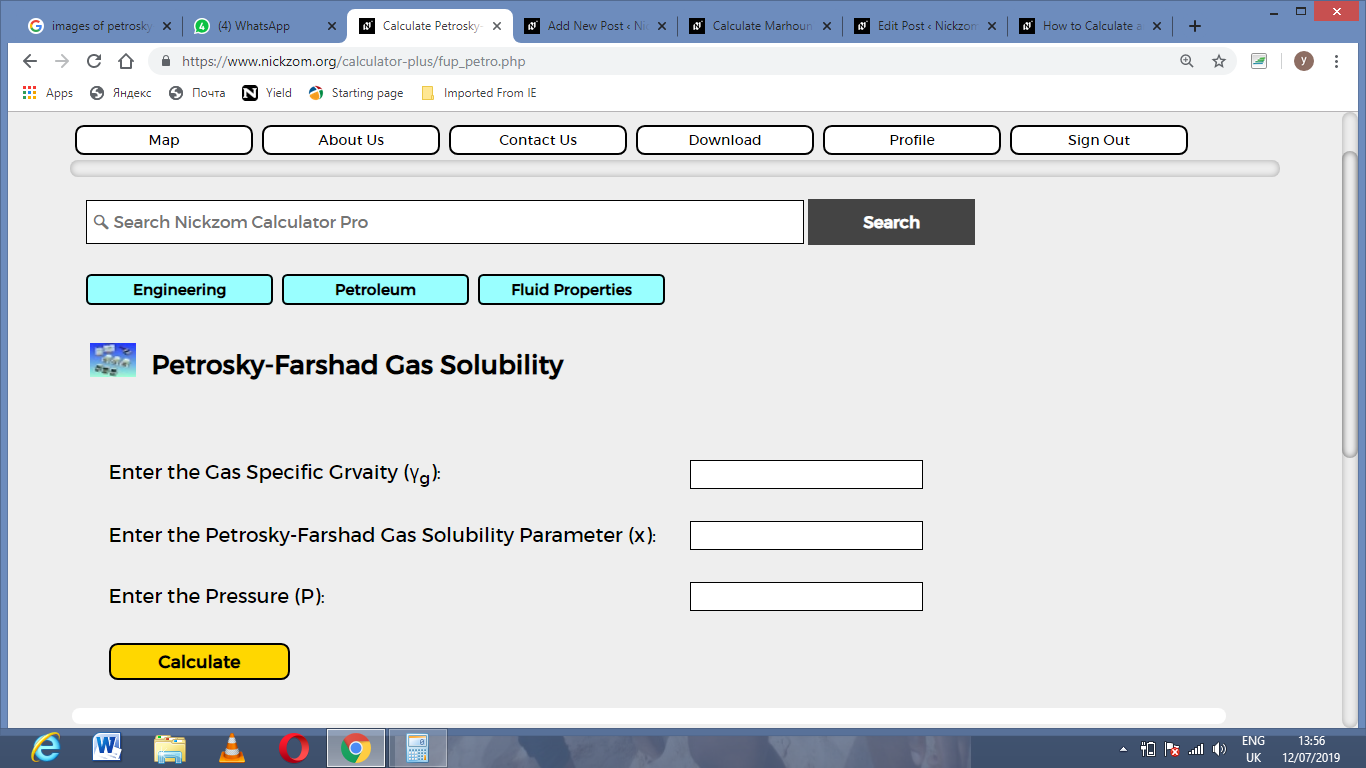Now, enter the values appropriately and accordingly for the parameters as required by the gas specific gravity (γg) is 16, petrosky-farshad gas solubility parameter (x) is 22 and pressure (P) is 80.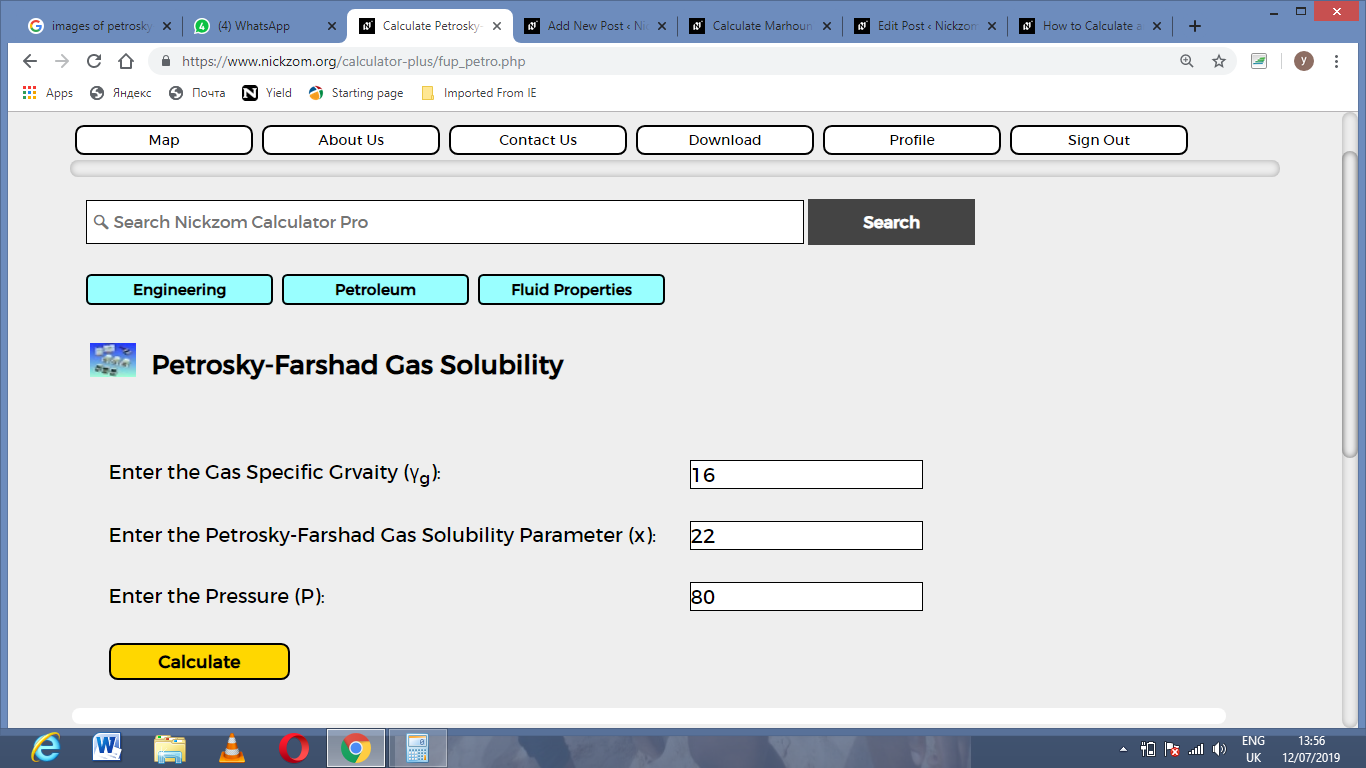Finally, Click on Calculate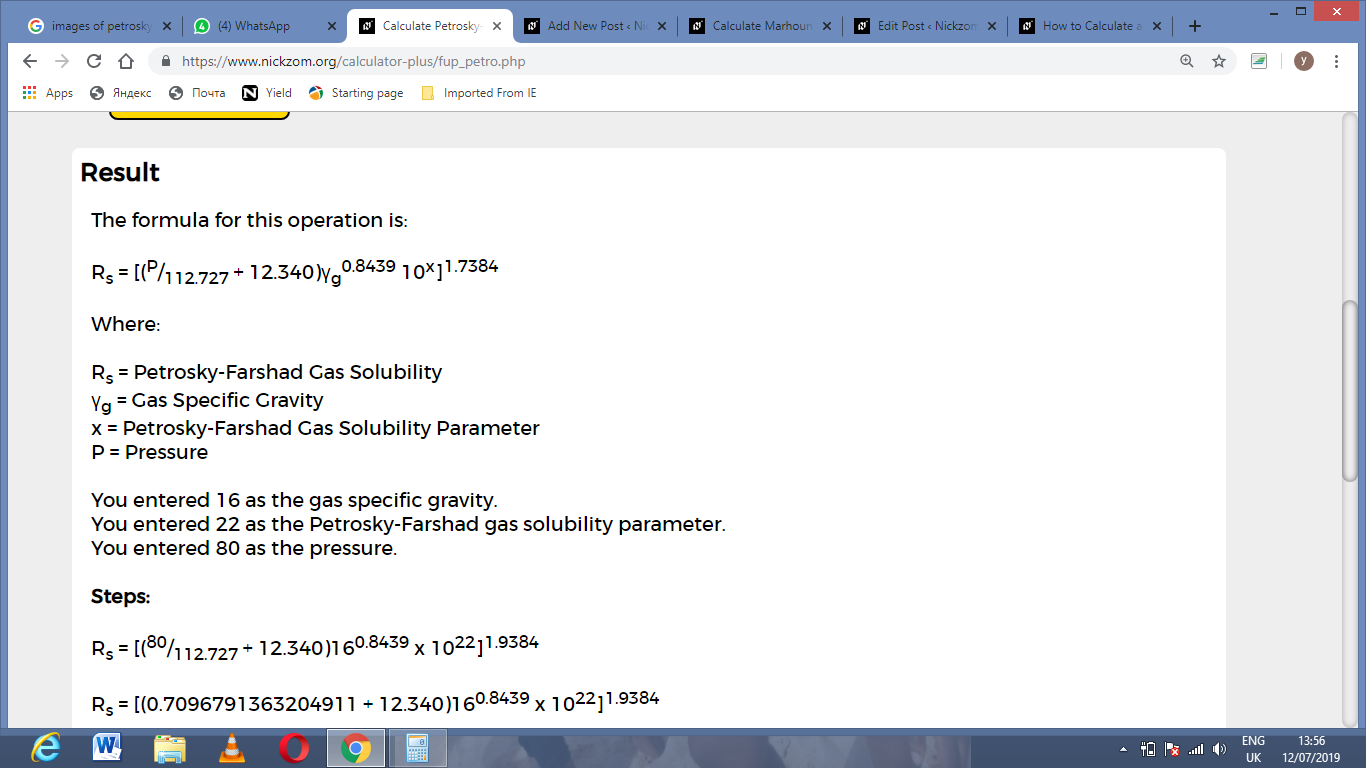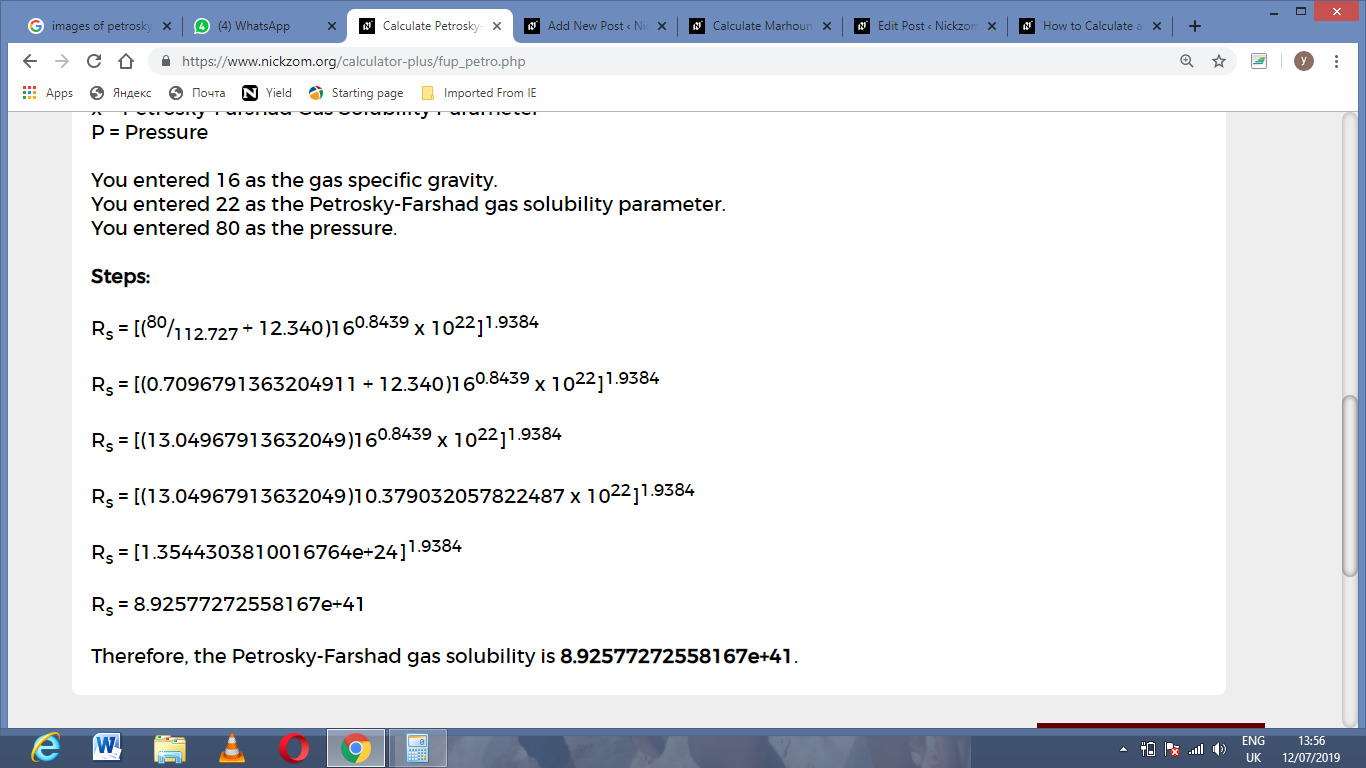As you can see from the screenshot above, Nickzom Calculator– The Calculator Encyclopedia solves for the petrosky-farshad gas solubility and presents the formula, workings and steps too.